# How to find sum of largest N numbers in Excel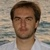by , updated on

Six fast and reliable ways to do sum of the highest N values in Excel.

Adding things up is one of the most popular activities both in the business world and in everyday life. No wonder that Microsoft Excel comes equipped with a number of inbuild function such as, SUM, SUMIF, and SUMIFS.

In some situations, however, you may need to sum only specific numbers in a range, say top 3, 5, 10 or n. That might be a challenge because Excel has no inbuilt function for this. But as always, there is nothing that would prevent you from constructing your own formulas :)

## SUM largest 2, 3, 5 or n numbers in a range

To sum top n numbers in a given array, the generic formula is:

SUM(LARGE(range, {1,2,3, …, n}))

For example, to get the sum of the largest 2 numbers in the range B2:B15, the formula is:

`=SUM(LARGE(B2:B15, {1,2}))`

To sum 3 largest numbers:

`=SUM(LARGE(B2:B15, {1,2,3}))`

To do a sum of 5 largest numbers:

`=SUM(LARGE(B2:B15, {1,2,3,4,5}))`

The screenshot below shows all the formulas in action: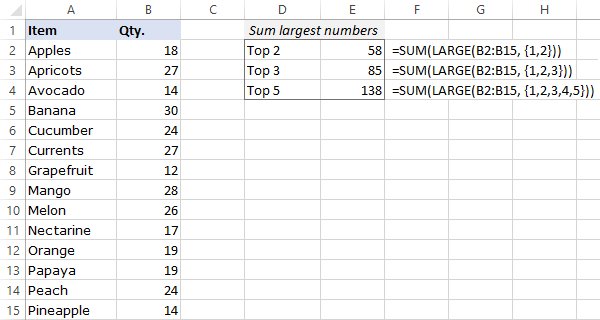If the values' ranks are input in predefined cells, you'd need to use a range reference for the k argument of LARGE. In this case, the solution must be entered as an array formula by pressing the Ctrl + Shift + Enter keys together. In Dynamic Array Excel (365 and 2021), this will also work as a regular formula.

For example, to get sum of the largest 3 adjacent or non-adjacent numbers whose ranks are in D2:D4, the formula is:

`=SUM(LARGE(B2:B15, D2:D4))`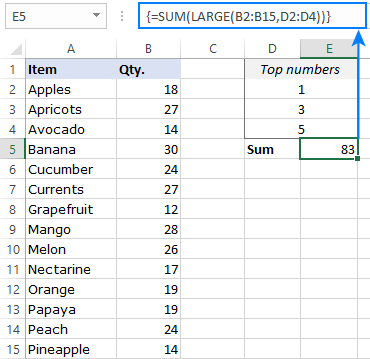Note. If there are two or more numbers that are tied for the last place, only the first number will be summed. For example, if the top 3 numbers are 10, 9 and 7, and the number 7 occurs in more than one cell, only the first occurrence of 7 will be added up.

How this formula works:

The core of the formula is the LARGE function that determines the Nth largest value in a given array. To get the top n values, an array constant such as {1,2,3} is supplied to the second argument:

`LARGE(B2:B15, {1,2,3})`

As the result, LARGE returns the top 3 values in the range B2:B15, which are 30, 28 and 27.

The SUM function takes it from there, adds up the 3 numbers, and outputs the total:

`=SUM({30,28,27})`

## Find sum of highest values with SUMPRODUCT

The SUMPRODUCT formula to return a sum of top N numbers is very much alike:

SUMPRODUCT(range, {1,2,3, …, n}))

The beauty of SUMPRODUCT is that it works with array constants and cell references equally well. That is, regardless of whether you use {1,2,3} or D2:D4 for k inside LARGE, a regular formula will work nicely in all versions of Excel:

`=SUMPRODUCT(LARGE(B2:B15, {1,2,3}))`

Or

`=SUMPRODUCT(LARGE(B2:B15, D2:D4))`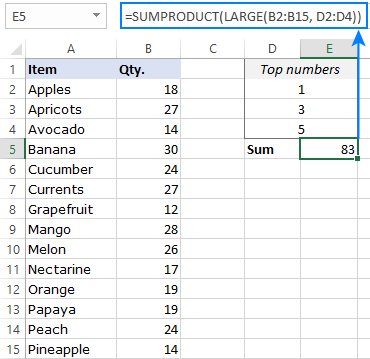## How to sum many top numbers

If you are looking to add up the top 10 or 20 numbers in a range, listing their positions in an array constant manually may be quite tiresome. In this case, you can construct an array automatically by using the ROW and INDIRECT functions:

SUMPRODUCT(LARGE(range, ROW(INDIRECT("1:n"))))
SUM(LARGE(range, ROW(INDIRECT("1:n"))))

Please remember that the SUM + LARGE combination only works as an array formula in Excel 2019 and lower, so use the Ctrl + Shift + Enter shortcut to complete it correctly.

For instance, to sum 10 highest numbers in the range, use one of these formulas:

`=SUM(LARGE(B2:B15, ROW(INDIRECT("1:10"))))`

Or

`=SUMPRODUCT(LARGE(B2:B15, ROW(INDIRECT("1:10"))))`

To make the formula more flexible, you can enter the number of items in some cell, say E2, and concatenate the cell reference inside INDIRECT:

`=SUM(LARGE(B2:B15, ROW(INDIRECT("1:"&E2))))`

Or

`=SUMPRODUCT(LARGE(B2:B15, ROW(INDIRECT("1:"&E2))))`

In Excel 365 and Excel 2021, where the dynamic array behavior is native for all functions, both SUM and SUMPRODUCT work perfectly as regular formulas: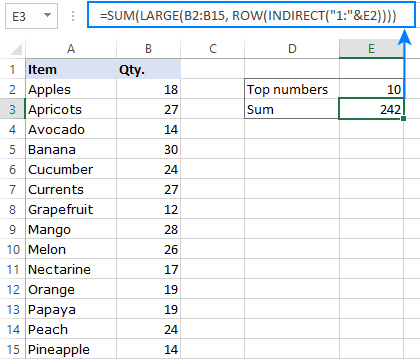## How to sum a variable number of largest values

When there is insufficient data in the source range, i.e. n is larger than the total number of items, the formulas discussed above would result in an error: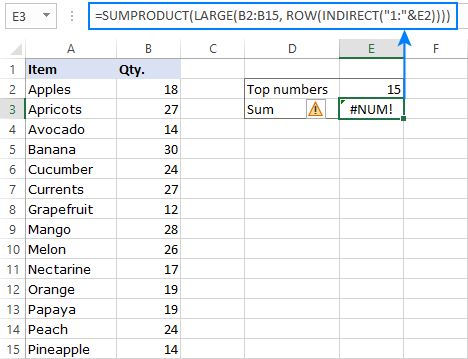To handle this scenario, choose one of these solutions:

### 1. Return the sum of n largest or all items

With this approach, you output a sum of all existing values in case the specified n is larger than the total number of items in the range.

First, we get a count of all the numbers with the help of COUNT, and then use MIN to return either n or the count, whichever is smaller:

`=SUMPRODUCT(LARGE(range, ROW(INDIRECT("1:"&MIN(n, COUNT(range))))))`

For our sample dataset, the formula takes this form:

`=SUMPRODUCT(LARGE(B2:B15, ROW(INDIRECT("1:"&MIN(E2, COUNT(B2:B15))))))`

Because the specified n (15) is higher than the count (14), all the numbers in B2:B15 are summed: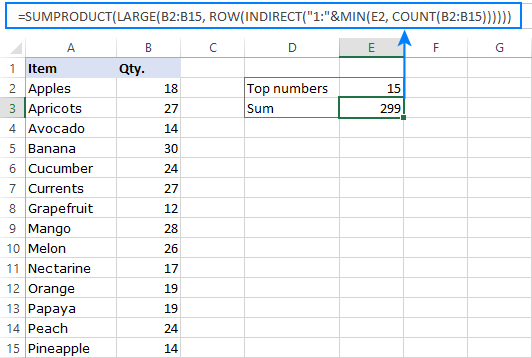### 2. Show a custom message if not enough data

To make it explicitly clear that n exceeds the total number of items in the list, you can display a custom message using the IFERROR function:

`=IFERROR(SUMPRODUCT(LARGE(B2:B15, ROW(INDIRECT("1:"&E2)))), "Insufficient data")`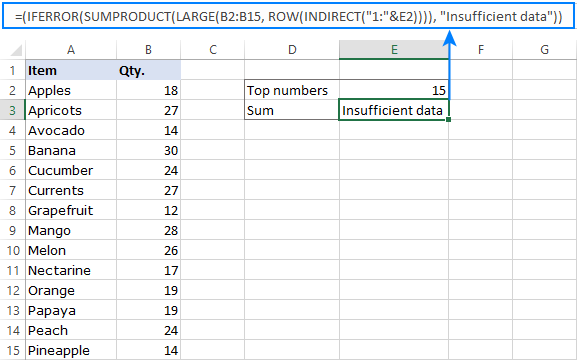## Sum largest numbers in Excel 365 and Excel 2021

Instead of constructing a cumbersome ROW + INDIRECT combination, the users of Excel 365 and Excel 2021 can employ the SEQUENCE function to generate a numeric array on the fly:

SUMPRODUCT(LARGE(range, SEQUENCE(n)))

Because SEQUENCE is only available in the new Dynamic Array Excel where the array behavior is native for all the functions, you can safely replace SUMPRODUCT with a more concise SUM:

SUM(LARGE(range, SEQUENCE(n)))

For example, to do sum of 5 largest numbers, use this compact and elegant formula:

`=SUM(LARGE(B2:B15, SEQUENCE(E2)))`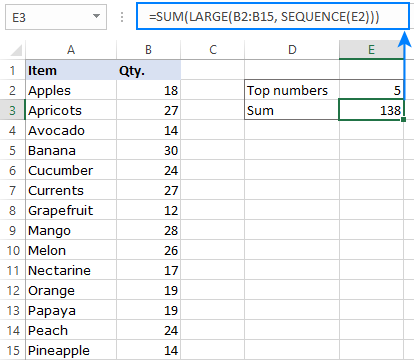## How to sum top n values in Excel table

Excel tables come equipped with a number of awesome features, which let you get a sum of top N values in 4 easy steps:

1. Convert a usual range to a table by pressing the Ctrl + T keys together.
2. On the Table Design tab, in the Table Style Options group, enable the Total Row feature.
3. In the header of the column containing your numbers, click the filter icon, and then click Number Filters > Top 10.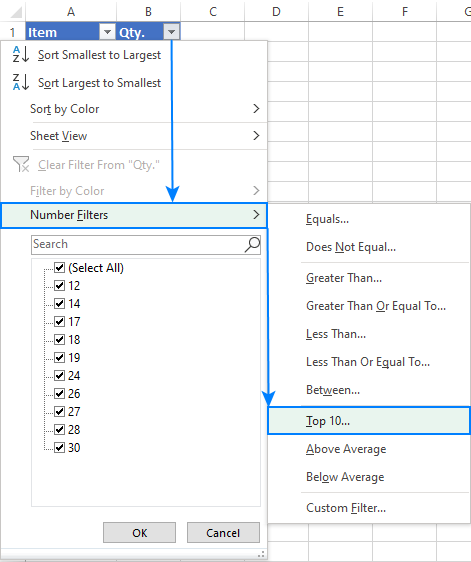4. In a pop-up dialog box, specify how many top items to display: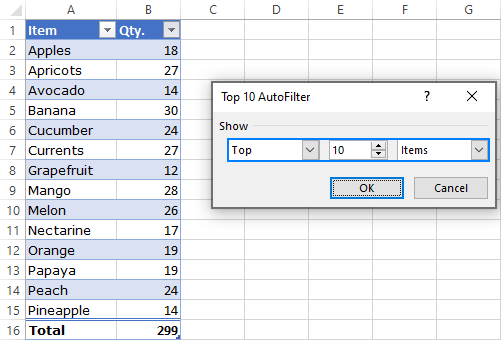That's it! Excel will immediately filter 10 top items (or whatever you choose) and calculate their total automatically.

To verify the result, let's compare the table's total with the result of our formula and make sure they are fully in line: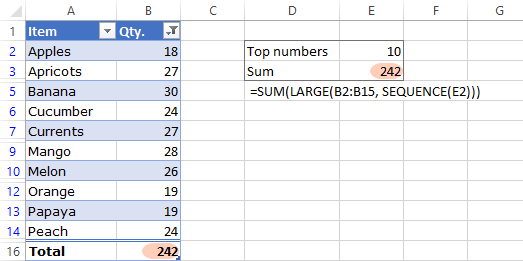These are the 6 effective ways to add up highest values in Excel. I thank you for reading and hope to see you on our blog next week!